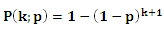# SQL Server geometric inverse distribution function

GEOMETRICINV

Updated: 31 July 2010

Use GEOMETRICINV to calculate the inverse of the cumulative distribution function of the geometric distribution.

The formula for the cumulative distribution function is:Syntax
SELECT [wctStatistics].[wct].[GEOMETRICINV] (
<@CDF, float,>
,<@P, float,>)
Arguments
@CDF
is the cumulative distribution function. @CDF is an expression of type float or of a type that implicitly converts to float
@P
is the probability of success. @P is an expression of type float or of a type that implicitly converts to float
Return Types
float
Remarks
·         @CDF must be greater than zero and less than or equal to one (0 < @CDF ≤ 1).
·         @P must be greater than zero and less than or equal to one (0 < @P ≤ 1).
Examples
Calculate the cumulative distribution function:
SELECT wct.GEOMETRICP(3,0.4)
This produces the following result
----------------------
0.8704

(1 row(s) affected)

Calculate the inverse:
SELECT wct.GEOMETRICINV(.8704,0.4)
This produces the following result
----------------------
3

(1 row(s) affected)

### SupportCopyright 2008-2023 Westclintech LLC         Privacy Policy        Terms of Service